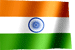RAMESH KRISHNA REDDY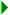SQLIntroduction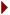DB2 SQL DDLDB2 SQL DMLDB2 SQL DML Part IIDB2 SQL DML Part III

DB2 SQL - DML (Data Manipulation Language) - Part III

In this part of the tutorial we will discuss about GROUP BY clause, HAVING clause and Functions in SQL.
Let us assume we have following tables defined on our database.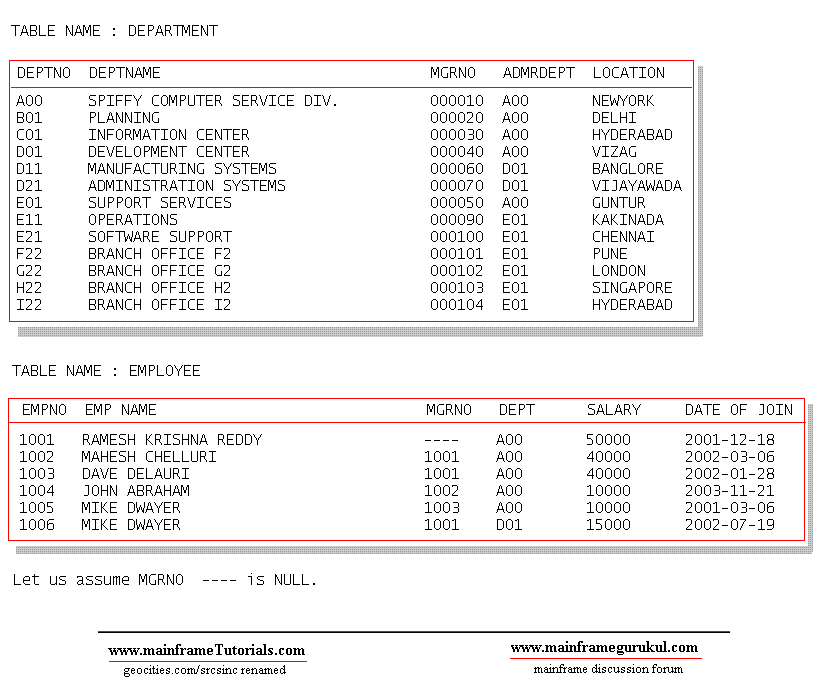GROUP BY & HAVING

One special use of GROUP BY is to associate an aggregate function
(especially COUNT; counting the number of rows in each group) with groups of rows.

Example 1 : Get all Departments with employees count from EMPLOYEE table.

```
SQL Query :

SELECT DEPT, COUNT(*) FROM EMPLOYEE
GROUP BY DEPT

Result :

-------   --------
DEPT
-------   --------
A00        5
D01        1
```
Example 2 : Get all Department names with employees > 4

```
SQL Query :

SELET DEPT, COUNT(*) FROM EMPLOYEE
GROUP BY DEPT
HAVING COUNT(*) > 4

Result :

-------   --------
DEPT
-------   --------
A00        5

```

 FUNCTIONS

```
A function is an operation denoted by a function name followed by one or more
operands which are enclosed in parentheses. The operands of functions are called
arguments. Most functions have a single argument that is specified by an
expression. The result of a function is a single value derived by applying the
function to the result of the expression.```
Following are the functions available in DB2 SQL.

Column Functions
AVG
COUNT
MAX
MIN
SUM

Scalar Functions
CHAR
COALESCE
DATE
DAY
DAYS
DECIMAL
DIGITS FLOAT
HEX
HOUR
INTEGER
LENGTH
MICROSECOND .
MINUTE
MONTH
SECOND
SUBSTR
TIME
TIMESTAMP
VALUE
VARGRAPHIC
YEAR

COLUMN FUNCTIONS

A column function is applied to set of values.

 AVG

```
The AVG function returns the average of set of numbers

Example : Get average salary from EMPLOYEE table.

SQL Query :   SELECT AVG(SALARY) FROM EMPLOYEE
Result  :  27500

NOTE : If the function is applied to an empty set, the result is a null value

COUNT

This function gives the number of rows for each group ( If we use GROUP BY clause) or total number
of records in the result set.

Example : Get the number of employees in all departments from EMPOYEE table.

SQL Query : SELECT COUNT(*)  FROM EMPLOYEE
Result : 6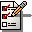While counting number of records , if you want to eliminate duplicates and null values use DISTINCT
EX. SELECT COUNT(DISTINCT EMP NAME) FROM EMPLOYEE - will give result 5, since we have records with
same name MIKE DWAYER

MAX / MIN

MAX function is used to get the maximum value from the set of values
MIN function is used to get the minimum value from the set of values

Example 1 : Get the maximum salary from EMPLOYEE table

SQL Query : SELECT MAX(SALARY) FROM EMPLOYEE
Result : 50000

Example 2 : Get the minimum salary from EMPLOYEE table

SQL Query : SELECT MIN(SALARY) FROM EMPLOYEE
Result : 10000

SUM

This function returns sum of a set of numbers. If the data type of the argument values is decimal,
the scale of the result is the same as the scale of the argument values and the precision of the
result depends on the precision of the argument values and the decimal precision option:

- If the precision of the argument values is greater than 15 or the DEC31 option
is in effect, the precision of the result is min(31,P+10), where P is the precision
of the argument values.

- Otherwise, the precision of the result is 15.

Exmaple :   Get the sum of all salaries from EMPOYEE table.

SQL Query  : SELECT SUM(SALARY) FROM EMPLOYEE
Result : 165000
```

SCALAR FUNCTIONS

A scalar function is applied to single value rather than a set of values.

 CHAR
```
This function returns the character representation of date, time, timestamp or decimal.

Syntax  -  CHAR(expression [,ISO] [,USA] [,EUR] [,JIS] [,LOCAL] )

Examples SQL Queries -   Let us assume TEMP is table contains one record.

SELECT CHAR(CURRENT_DATE)
FROM TEMP
Result :  2005-12-17

SELECT CHAR(CURRENT_DATE,USA)
FROM TEMP
Result : 12/17/2005

SELECT CHAR(CURRENT_TIME,USA)
FROM TEMP
Result : 02:12 PM

SELECT CHAR(CURRENT_TIME)
FROM TEMP
Result : 14.12.43

DATE

The DATE function returns a date. if the argument is null, the result is the null value.
If the argument is a timestamp, the result is the date part of the timestamp.
If the argument is a date, the result is that date.
If the argument is a number, the result is the date that is n-1 days after
January 1, 0001, where n is the integral part of the number.

Example SQL Query :
SELECT DATE(CURRENT_TIMESTAMP)
FROM  TEMP

Result : 2004-11-17

DAY

This function returns the day part of the argument. If the argument is null, the result is the null value.

Example :	Assume that DATE1 and DATE2 are DATE columns in the same
table. Assume also that for a given row in this table, DATE1 and DATE2 represent
respectively the dates 15 January 2000 and 31 December 1999. Then, for the
given row:

DAY(DATE1 - DATE2)
returns the value 15.

DAYS

This function returns the integer representation of given date. This function caluculate the number of
days from January 1, 0001 to the argument date. If the argument is null, the result is the null value.

Example SQL Query :
SELECT DAYS('2003-11-21')
FROM TEMP
Result :  731540

DECIMAL

This function returns decimal represenation of given value.

Syntax - DECIMAL(expression [,integer1] [,integer2] )

The data type of the result is DECIMAL(p,s), where p and s are the second and
third arguments. ( integer1, integer2 ). expression can be character string or
a number.

Represent the average salary of the employees in EMPLOYEE-2 table as an
10-digit decimal number with two of these digits to the right of the decimal point.

Example SQL Query :
SELECT DECIMAL(AVG(SALARY),8,2)
FROM  EMPLOYEE

DIGITS

The DIGITS function returns a character string representation of its argument.
The argument must be an integer or a decimal number. if the argument is null,
the result is the null value.

Example :   Assume that BALANCE has the data type DECIMAL(6,2), and that
one of its values is -6.28. Then, for this value:

SELECT DIGITS(BALANCE) FROM BANK
Return value will be  '000628'.

The result is a string of length six (the precision of the column) with leading zeros
padding the string out to this length. Neither sign nor decimal point appear in the
result.

FLOAT - HEX - HOUR - INTEGER

FLOAT	-	The FLOAT function returns a floating-point representation of its argument.
if the argument is null, the result is the null value.

HEX	-	The HEX function returns a hexadecimal representation of its argument.
if the argument is null, the result is the null value.

HOUR	-	This function is to get the hour part from time or timestamp
if the argument is null, the result is the null value.

INTEGER	-	This function returns an integer representation of given value.
if the argument is null, the result is the null value.

LENGTH

LENGTH function returns the length of the given argument.
if the argument is null, the result is the null value.

Example :  Assume that EMPNAME is a VARCHAR(14) column that contains
'RAMESH' for employee 200. The following SQL query:

SELECT LENGTH(EMPNAME)
FROM EMPLOYEE-2
WHERE EMPNO = '200'

This query returns value 6

MICROSECOND

The MICROSECOND function returns the microsecond part of its argument. The argument must be a timestamp or timestamp
duration. The result of the function is a large integer. if the argument is null, the result is the null value.

MINUTE

The MINUTE function returns the minute part of its argument. The argument must be a time, timestamp, time duration, or
timestamp duration. The result of the function is a large integer. if the argument is null, the result is the null value.

MONTH

The MONTH function returns the month part of its argument. The argument must be a date, timestamp, date duration, or
timestamp duration. The result of the function is a large integer. If the argument is null, the result is the null value.

Example : Select all rows in the table EMPLOYEE-2 for employees who
were born in May:  SQL Query :

SELECT FROM EMPLOYEE-2
WHERE MONTH(BIRTHDATE) = 5;

SECOND

SECOND function returns the seccond part of time or timestamp. If argument is null
Result will be null.

Example : If APP-RECEIVED timestamp is  2004-10-21-11.09.20.000012'

SUBSTR

SUBSTR function returns the part of given string. If the argument is null, function returns null value.

Syntax - SUBSTR(string,start [,length])

Here string is the input string, start - is the starting position of substring in the given string.
length - length of the substring. If this parameter is ommited the result will be number of characters
starting from position specified in "start" parameter till the end of the string.

Example : Let us assume EMPNAME contains "RAMESH" , we wan to get first three characters
from the EMPNAME.

SUBSTR(EMPNAME,1,3) returns the first 3 characters which is "RAM"

TIME - TIMESTAMP

The TIME function returns a time derived from its argument. The argument must be a timestamp, a time, or a valid
string representation of a time. If the argument is null, the result is the null value.

The TIMESTAMP function returns a timestamp derived from its argument or arguments. The rules for the arguments
depend on whether the second argument is specified.

If both arguments are specified, the first argument must be a date or a valid string representation of a date and
the second argument must be a time or a valid string representation of a time.

Syntax - TIMESTAMP(expression [,expression])

VALUE

VALUE function returns the value of given value. COALESCE can be
used as a synonym for VALUE.

Syntax - VALUE(expression [, expression ] )

If argument1 is null, This function returns argument2 value.
Argument1, Argument2 must be of same type.

Example : Assume that MARK1 and MARK2 are columns in table
STUDENT, and nulls are allowed in MARK1 column. Select all
the rows in STUDENT for which MARK1 + MARK2 > 120, assuming a value of 0
for MARK1 when MARK1 is null.

SQL Query :

SELECT * FROM STUDENT
WHERE VALUE(MARK1,0) + MARK2 > 100;

VARGRAPHIC

The VARGRAPHIC function returns a graphic string representation of a character string. if the argument is null,
the result is the null value.

YEAR

The year function retuns the year part of its argument. If the argument is null, result will be null value.

Example :  Get the all employees who are born in 1977

SQL Query :

SELECT * FROM EMPLOYEE
WHERE YEAR(BIRTH_DATE) = 1977

```

SQL / DB2 UNIVERSAL DATABASE / DB2 DATABASE / SQL / DB2 / DB2 DATABASE / SQL / DB2 / DB2 DATABASE

Drona Tutorials - DB2 Tutorials